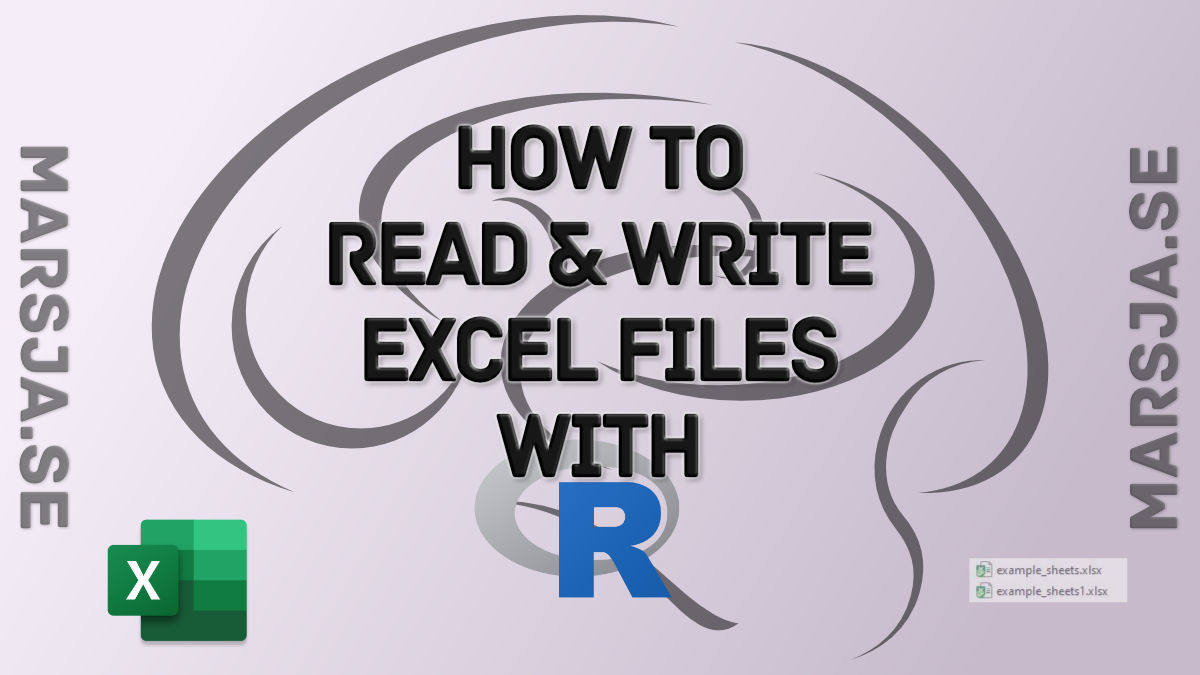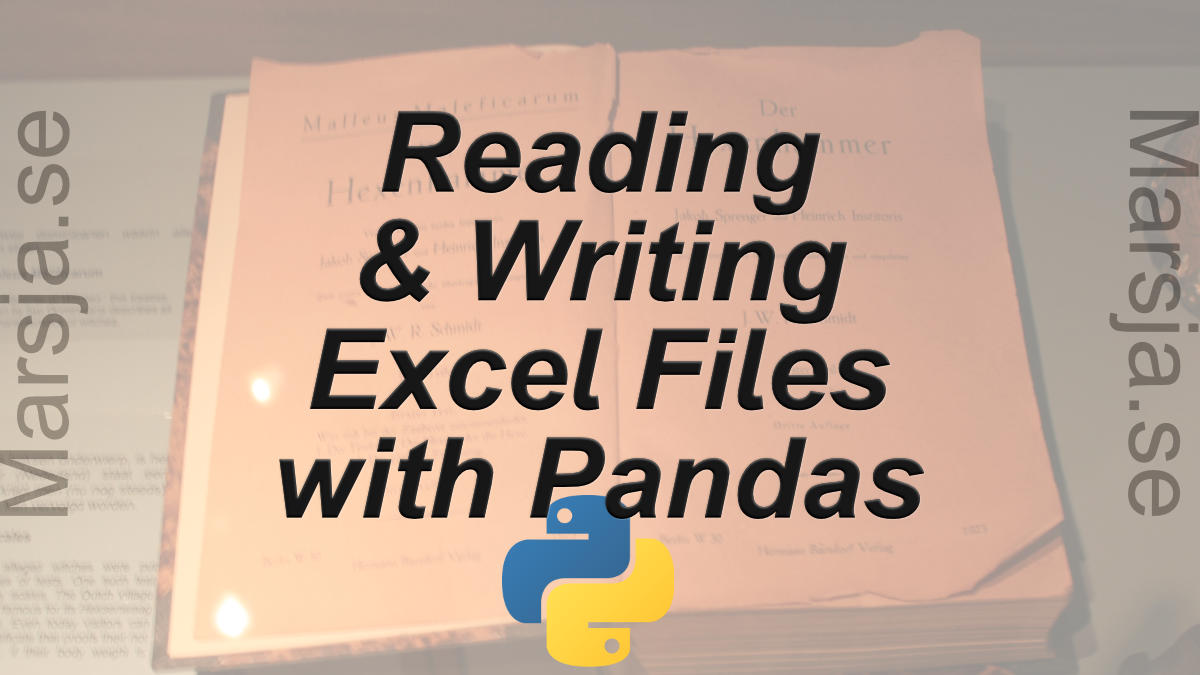## Seaborn Line Plots: A Detailed Guide with Examples (Multiple Lines)

In this Python data visualization tutorial, we will learn how to create line plots with Seaborn. First, we’ll start with the simplest example (with one line) and then we’ll look at how to change the look of the graphs, and how to plot multiple lines, among...## R Excel Tutorial: How to Read and Write xlsx files in R

In this tutorial, we will learn how to work with Excel files in R statistical programming environment. It will provide an overview of how to use R to load xlsx files and write spreadsheets to Excel. In the first section, we will go through, with examples, how to use R...## Pandas Excel Tutorial: How to Read and Write Excel files

In this Pandas Excel tutorial, we will learn how to work with Excel files in Python. It will provide an overview of how to use Pandas to load xlsx files and write spreadsheets to Excel. In the first section, we will go through, with examples, how to use Pandas...## OpenSesame Tutorial – How to Create a Flanker Task

In this post, you are going to learn how to create a simple experiment using the free experiment building software OpenSesame. As I have previously written about, OpenSesame, is an application, based on Python, for creating Psychology, Neuroscience, and Economics...

## R from Python – an rpy2 tutorial

Rpy2 Tutorial: How to Run R in Python In this post, we are going to answer the question can you run R in Python? Of course, the answer is yes!; we can use the Python package rpy2. This package offers a Python interface to R. In this tutorial, we will learn how to...